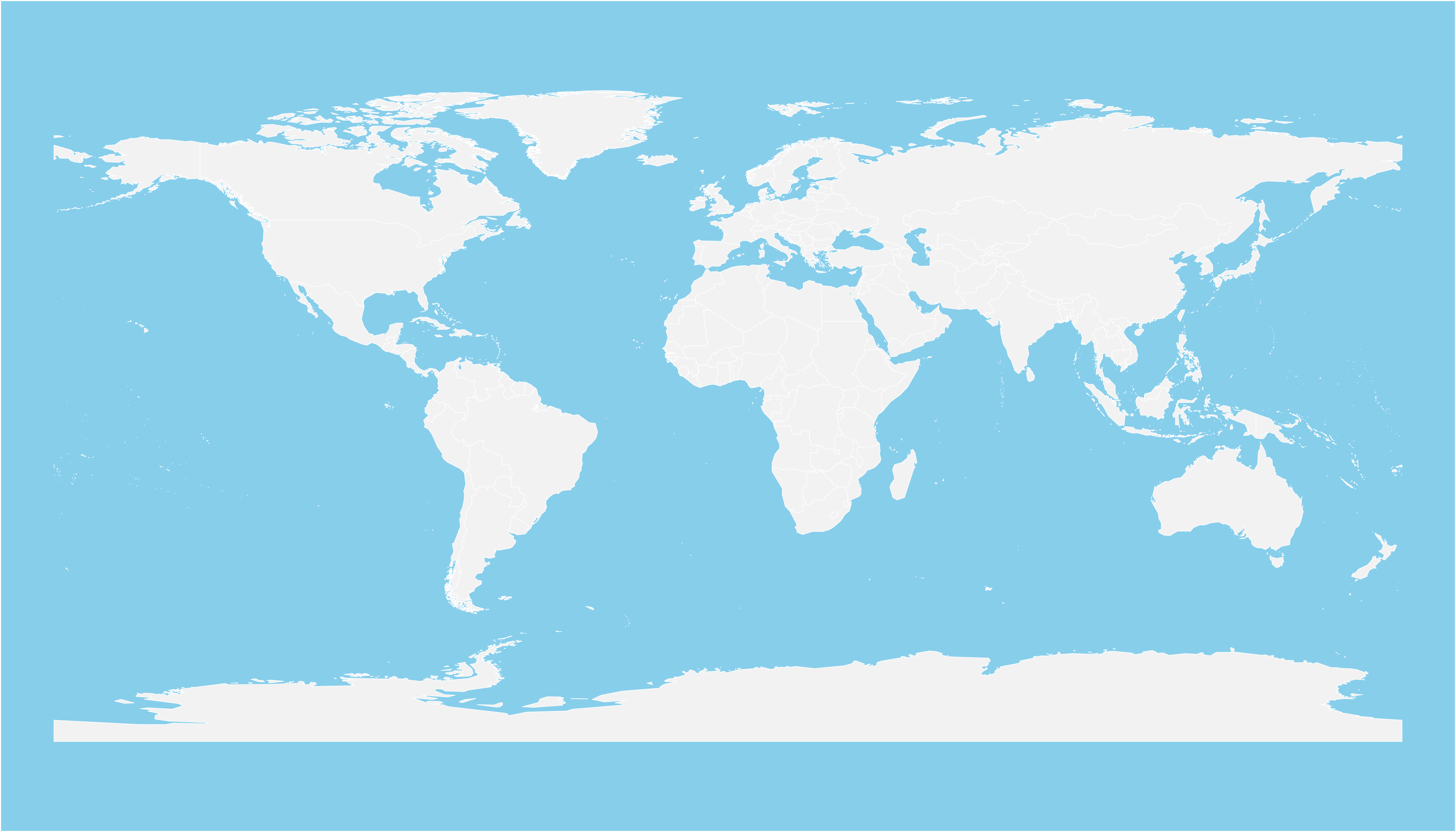# Open and Plot Shapefiles in R

Shapefiles are a common way to store geospatial data. This post explains how to read it with `R` and the `rgdal` package, and how to plot it in base `R` or with `ggplot2`.

If you did not find the geospatial data you need in existing R packages (see the map section), you need to find this information elsewhere on the web.

Usually, you will find it as a shape file format. This format is composed by several files that you need to keep together in the same folder.

Note: if you found a `.geoJSON` file, read this post instead.

You need to dig the internet to find the shape file you are interested in. For instance, this URL will redirect you to a zipped shape file containing the worl boundaries.

``````# Download the shapefile. (note that I store it in a folder called DATA. You have to change that if needed.)
# You now have it in your current working directory, have a look!

# Unzip this file. You can do it with R (as below), or clicking on the object you downloaded.
system("unzip DATA/world_shape_file.zip")
#  -- > You now have 4 files. One of these files is a .shp file! (TM_WORLD_BORDERS_SIMPL-0.3.shp)``````

# Read it with `rgdal`

The `rgdal` package offers the `readOGR()` function that allows to read shapefile using the following syntax.

As a result you get a geospatial object (`my_spdf` here) that contains all the information we need for further mapping. Please try th following command to understand how this object works:

• `summary(my_spdf)`: tells you the max and min coordinates, the kind of projection in use
• `length(my_spdf)`: how many regions you have
• `head(my_spdf@data)`: the firs few rows of the data slot associated with the regions
``````# Read this shape file with the rgdal library.
library(rgdal)
dsn= paste0(getwd(),"/DATA/world_shape_file/") ,
layer="TM_WORLD_BORDERS_SIMPL-0.3",
verbose=FALSE
)

# -- > Now you have a Spdf object (spatial polygon data frame). You can start doing maps!``````

# Plot it with `base R`

The basic `plot()` function knows how to plot a geospatial object. Thus you just need to pass it `my_spdf` and add a couple of options to customize the output.``````# Basic plot of this shape file:
par(mar=c(0,0,0,0))
plot(my_spdf, col="#f2f2f2", bg="skyblue", lwd=0.25, border=0 )``````

# Plot it with `ggplot2`

It is totally possible (and advised imo) to build the map with ggplot2. However, ggplot2 takes as input data frames, not geospatial data.

`my_spdf` thus needs to be transformed using the `tidy()` function of the `broom` package. The `region` argument of this function expect one of the column name if the @data slot. It will be the region name in the new dataframe.

Once the data frame is created, it is plotted using the `geom_polygon()` function as described below.``````# 'fortify' the data to get a dataframe format required by ggplot2
library(broom)
spdf_fortified <- tidy(my_spdf, region = "NAME")

# Plot it
library(ggplot2)
ggplot() +
geom_polygon(data = spdf_fortified, aes( x = long, y = lat, group = group), fill="#69b3a2", color="white") +
theme_void() ``````

Related chart types

## Contact

This document is a work by Yan Holtz. Any feedback is highly encouraged. You can fill an issue on Github, drop me a message on Twitter, or send an email pasting yan.holtz.data with gmail.com.